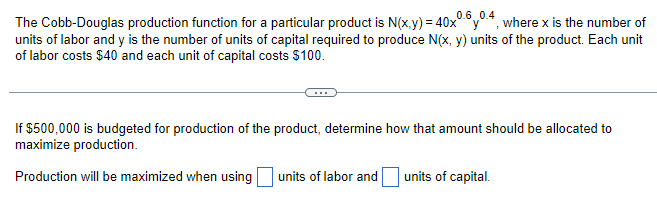Home / Expert Answers / Calculus / the-cobb-douglas-production-function-for-a-particular-product-is-mathrm-n-x-y-40-x-0-6-y-pa407

# (Solved): The Cobb-Douglas production function for a particular product is $$\mathrm{N}(x, y)=40 x^{0.6} y^ ...The Cobb-Douglas production function for a particular product is \( \mathrm{N}(x, y)=40 x^{0.6} y^{0.4}$$, where $$x$$ is the number of units of labor and $$y$$ is the number of units of capital required to produce $$N(x, y)$$ units of the product. Each unit of labor costs $$\ 40$$ and each unit of capital costs $$\ 100$$. If $$\ 500,000$$ is budgeted for production of the product, determine how that amount should be allocated to maximize production. Production will be maximized when using units of labor and units of capital.

We have an Answer from Expert